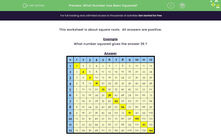# Find Simple Square Roots of Numbers

In this worksheet, students will work out simple square roots.Key stage:  KS 2

Curriculum topic:   Number: Multiplication and Division

Curriculum subtopic:   Recognise Square/Cube Numbers

Popular topics:   Square Numbers worksheets

Difficulty level:#### Worksheet Overview

Can you remember what we mean by a square  number?A square number is made when we multiply a number by itself, eg. 3 x 3 = 9 or 10 x 10 = 100.

9 and 100 are square numbers.

The number that we multiplied to get a square number is the square root. So, the square root of 9 is 3 and the square root of 100 is 10.

Does that make sense?This activity is all about square roots.

Example

What number squared gives the answer 36? Or, we could ask, what is the square root of 36?In the grid above, square numbers are shown in yellow.

Find 36 and see what was multiplied together to get that answer.

6 x 6 = 36

6 squared is 36 and the square root of 36 is 6.

Let's make a start on the questions now.

If you want to look at this grid again, click on the red help button on the screen at any point during the activity.

### What is EdPlace?

We're your National Curriculum aligned online education content provider helping each child succeed in English, maths and science from year 1 to GCSE. With an EdPlace account you’ll be able to track and measure progress, helping each child achieve their best. We build confidence and attainment by personalising each child’s learning at a level that suits them.

Get started